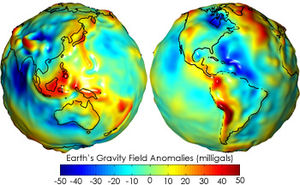Chinese Geophysical Data Center, World Data Center. 世界数据中心 中国地球物理分中心# Knowledge

•Geomagnetic
•Seismic wave
•Gravity of Earth
•Electromagnetic radiation
•Magnetosphere
•Sunspot Number
•Space Weather
•Aurora

# Gravity of EarthThe gravity of Earth, which is denoted by g, refers to the acceleration that the Earth imparts to objects on or near its surface. In SI units this acceleration is measured in metres per second squared (in symbols, m/s2) or equivalently in newtons per kilogram (N/kg). It has an approximate value of 9.81 m/s2, which means that, ignoring the effects of air resistance, the speed of an object falling freely near the Earth's surface will increase by about 9.81 metres (32.2 ft) per second every second. This quantity is sometimes referred to informally as little g (in contrast, the gravitational constant G is referred to as big G).

There is a direct relationship between gravitational acceleration and the downwards force (weight) experienced by objects on Earth, given by the equation F = ma (force = mass × acceleration). However, other factors such as the rotation of the Earth also contribute to the net acceleration.

The precise strength of Earth's gravity varies depending on location. The nominal "average" value at the Earth's surface, known as standard gravity is, by definition, 9.80665 m/s2 (about 32.1740 ft/s2). This quantity is denoted variously as gn, ge (though this sometimes means the normal equatorial value on Earth, 9.78033 m/s2), g0, gee, or simply g (which is also used for the variable local value).

## Variation in gravity and apparent gravity

A perfect sphere of uniform density, or whose density varies solely with distance from the centre (spherical symmetry), would produce a gravitational field of uniform magnitude at all points on its surface, always pointing directly towards the sphere's centre. The Earth is not a perfect sphere, but is slightly flatter at the poles while bulging at the Equator: an oblate spheroid. There are consequently slight deviations in both the magnitude and direction of gravity across its surface. The net force (or corresponding net acceleration) as measured by a scale and plumb bob is called "effective gravity" or "apparent gravity". Effective gravity includes other factors that affect the net force. These factors vary and include things such as centrifugal force at the surface from the Earth's rotation and the gravitational pull of the Moon and Sun.